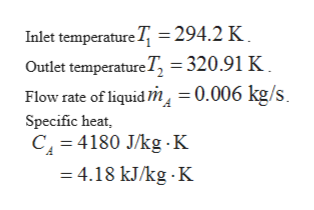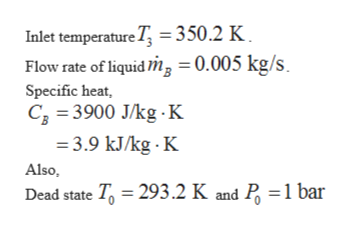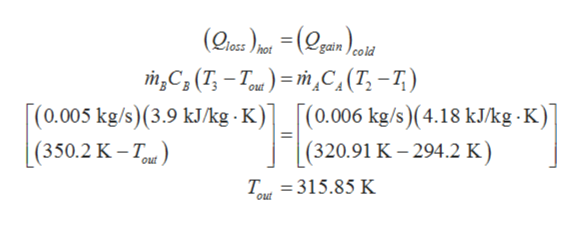# A heat exchanger is being investigated as a waste heat recovery device. A heat exchanger is common device for using a hot fluid to heat a cold fluid without the fluids mixing.  The following information is known.  The cold fluid stream of liquid A enters at 294.2 K and leaves the device at a temperature of 320.91 K.  Liquid A flows at a rate of 0.006 Kg/s and has a specific heat of 4180 J/(Kg K).  Liquid B enters the device at a temperature of 350.2 K.  Liquid B flows at a rate of 0.005 Kg/s and has a specific heat of 3900 J/(Kg K  The dead state is at 293.2 K and 1 bar.  The device is adiabatic and the pressure losses are neglected in this problem.  Both liquids are incompressible materials. Using the first law determine the exit temperature of Liquid B.Calculate the rate of exergy destroyed in the heat exchangerCalculate the second law efficiency for this device. The exergy product is the exergy increase in liquid A and the exergy input is the exergy change in liquid B.If the exiting liquid B is not used for any useful purpose, calculate the exergy destroyed associated with it.

Question
61 views
1. A heat exchanger is being investigated as a waste heat recovery device. A heat exchanger is common device for using a hot fluid to heat a cold fluid without the fluids mixing.  The following information is known.  The cold fluid stream of liquid A enters at 294.2 K and leaves the device at a temperature of 320.91 K.  Liquid A flows at a rate of 0.006 Kg/s and has a specific heat of 4180 J/(Kg K).  Liquid B enters the device at a temperature of 350.2 K.  Liquid B flows at a rate of 0.005 Kg/s and has a specific heat of 3900 J/(Kg K  The dead state is at 293.2 K and 1 bar.  The device is adiabatic and the pressure losses are neglected in this problem.  Both liquids are incompressible materials.

1. Using the first law determine the exit temperature of Liquid B.
2. Calculate the rate of exergy destroyed in the heat exchanger
3. Calculate the second law efficiency for this device. The exergy product is the exergy increase in liquid A and the exergy input is the exergy change in liquid B.
4. If the exiting liquid B is not used for any useful purpose, calculate the exergy destroyed associated with it.
check_circle

Step 1

Since you have posted a question with multiple subparts, we will solve the first three subparts, i.e. 1, 2, and 3 for you out of the 4 asked. To get the remaining subparts 4 solved, please re-post the question with the remaining subparts.

Consider the case for cold fluid.

Here,help_outlineImage TranscriptioncloseInlet temperature T = 294.2 K Outlet temperature T, = 320.91 K Flow rate of liquid in = 0.006 kg/s Specific heat, C 4180 J/kg K =4.18 kJ/kg K fullscreen
Step 2

Consider the case for hot fluid.

Here,help_outlineImage TranscriptioncloseInlet temperature T 350.2 K Flow rate of liquid m = 0.005 kg/s Specific heat, C 3900 J/kg K 3.9 kJ/kg K Also, Dead state T 293.2 K and P =1 bar fullscreen
Step 3

1.

Use the heat balanc...help_outlineImage Transcriptionclosegain loss hot mC3(T-T)mc,(T,-7) (0.005 kg/s)(3.9 k/kg. K)[(0.006 kg/s)(4.18 kJ/kg -K)] (320.91 K - 294.2 K out [(350.2 K -T) Out T 315.85 K out fullscreen

### Want to see the full answer?

See Solution

#### Want to see this answer and more?

Solutions are written by subject experts who are available 24/7. Questions are typically answered within 1 hour.*

See Solution
*Response times may vary by subject and question.
Tagged in

### Heat Transfer